Next: Parenthesis on the Minimal Up: Examples Previous: Unitarity Limit

## The Intermediate Vector Boson Hypothesis

This is the intention to formalize a theory of the weak interactions involving a dimensionless coupling constant and a particle working as a mediator of the weak interaction.

It is proposed to replace the Fermi current-current Lagrangian by the Lagrangian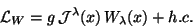(115)

which involves the field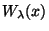corresponding to a charged vector boson - the mediator of the weak interaction - and the (semi) weak coupling constant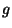. The Lagrangian (117) gives place, for example in the reaction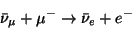(116)

to the Born term in the Figure 13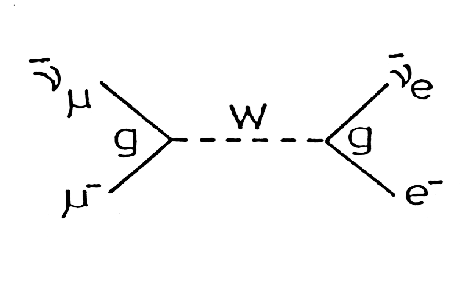instead of the point Fermi coupling.

In this way, the couplings of the example are written as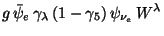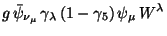Bearing in mind that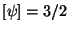and that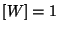there results that the (semi) weak constantis dimensionless as it was requested. On the other hand, the very short range of the weak interactions necessarily implies that the intermediate boson must be massive. Remembering the expression for the propagator of a vector boson with mass, it results for the amplitude of the process of the example: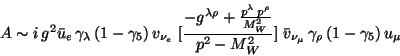(117)

where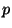is the momentum of the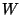boson.

It must be noticed that for low momentum regions, that is to say, when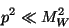(118)

from the amplitude (119) and the corresponding expression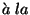Fermi it is concluded that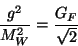(119)

This introduction shows that a model of weak interactions which bears a strong similitude with electrodinamics can be posed. Nevertheless, there are fundamental differences:

ex2html_comment_mark>60 0)sem

<
the bearer of the weak interaction, in contrast with the photon, is massive. As a consequence the contribution of the longitudinal polarization component of W will appear as can be seen in the propagator of (119).

<
the bearer of the weak interaction, in contrast with the photon, is a charged boson. Then the conservation of the electric charge will not make appear certain contributions equivalent to the ones that the photon allows in electrodynamics.

<
the weak current, in contrast with the electromagnetic, is not conserved.

Due to these distinctive characteristics it can immediately be observed that the theory of the intermediate boson of the weak interactions leads to difficulties similar to the ones presented by the model of point interaction. In other words, the higher order perturbative corrections of the theory cannot be calculated due to divergencies which continue to turn worse. The Lagrangian (117) has therefore the same phenomenological status than the Fermi type. In fact, the propagator of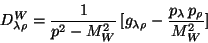(120)

behaves asymptotically as a constant. Due to this reason, in a contribution like the one in Figure 14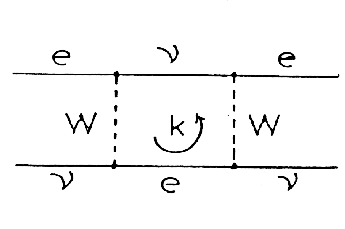one has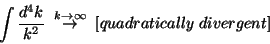(121)

It must be observed that in the equivalent contribution of the quantum electrodynamics pictured in Figure 15,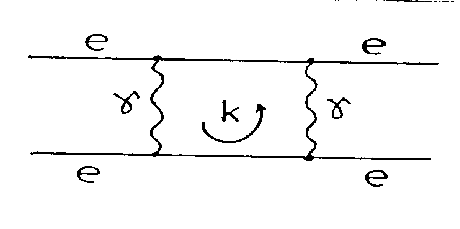due to the fact that the photon has no mass, there appears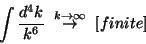(122)

It is clear, then, that the renormalization of the theory with intermediate boson based on the Lagrangian (117) is hopeless. In other words, the previous discussion indicates that the limit imposed by the unitarity to the cross section of a given process, via the optical theorem, will surely be violated by the massive intermediate vector boson model.

It should be noticed that the contribution of the term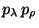in the propagator (122) is zero if the electron mass can be neglected due to the Dirac equation since these momenta multiply the lepton currents (see the amplitude (119)). Nevertheless the presence of a massivecauses new difficulties. Consider the process (see Figure 16)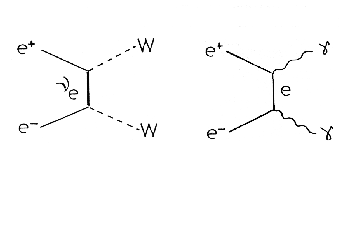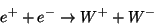(123)

clearly analogous to the QED one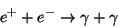(124)

The difference is that there is no longitudinal polarized photon, whereas W, being massive, implies both longitudinal and transverse polarization states. In fact, the cross section for transverseis well behaved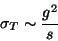(125)

while the longitudinal cross section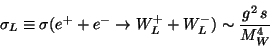(126)

clearly violates the unitarity limit.

Therefore, theLagrangian (117) has the same phenomenological status as the Fermi one. It gives rise to a non- renormalizable theory. On the contrary, QED is renormalizable. This important property is due to the fact that the photon is massless that in turn inhibits the longitudinal polarization state. This fact is directly related to the property of gauge invariance of electromagnetism.

In conclusion, it is necessary to implement gauge invariance in the weak interaction theory. This is not an easy task because there are clear differences with QED: while the photon is neutral and massless, theboson is charged and massive. Ability is needed.

It seems important to provide a cancellation mechanism between badly behaved amplitudes inspired in gauge invariant requierements.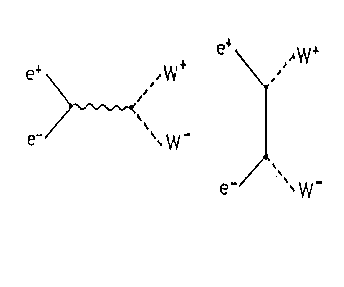Let us indicate the plausibility of these arguments analysing an example. We consider once more the annihilation reaction (125) that as it was established above, is ill behaved for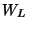. Using known (before 1970) particles, one can draw only the two graphs of Figure 17.

No cancellation is possible for the right electron state. Then, a new third graph is needed. One possibility is the one in Figure 6.4.

This graph includes a neutral weak boson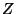that has to couple to both the right and the left states of the electron. This implies an electron neutral weak current and a precise relation between electromagnetism and neutral weak interactions just to guarantee the cancellation we were looking for. This scheme is correctly formalized by the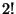model that we treat below.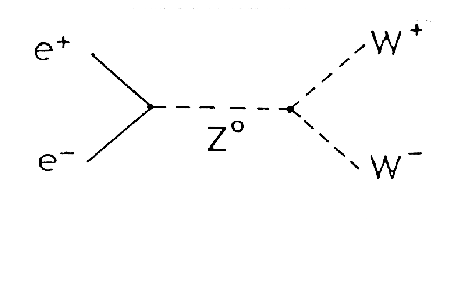Next: Parenthesis on the Minimal Up: Examples Previous: Unitarity Limit
root 2001-01-22